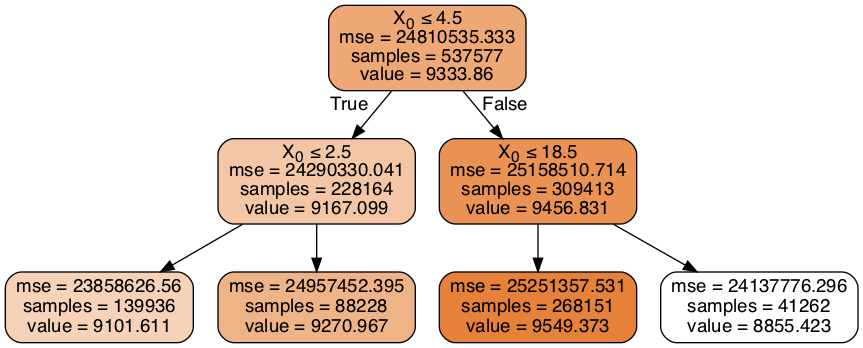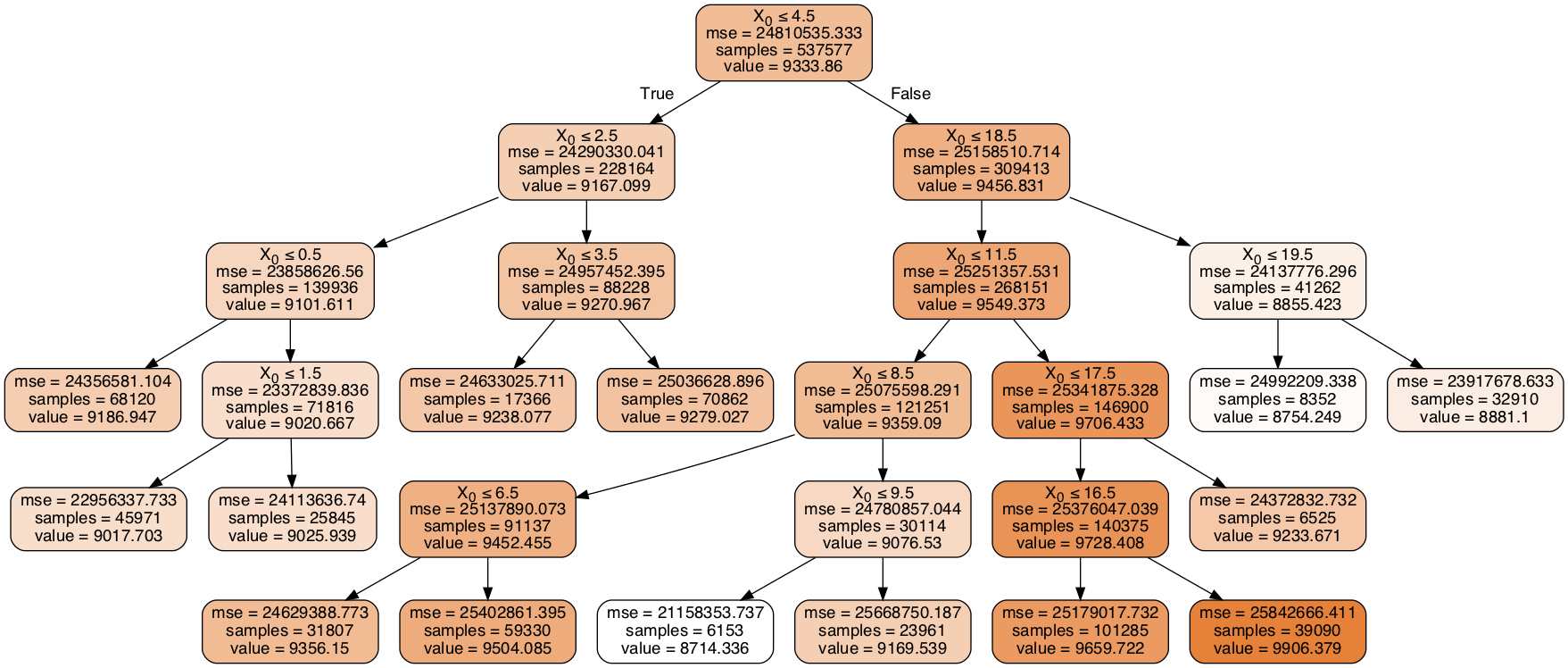Working with Categorical Variables with Multiple Levels: Python, Scikit-Learn, Multiple Correspondence Analysis

Posted on Mon 31 December 2018 in postsWorking with categorical variables that have a small number of classes (levels) can be a pleasant surprise from a data cleaning aspect for the data scientist/analyst just trying to get to next phase of their analysis. But sooner or later that one column with an unwieldy amount of classes will come along and slap you upside the head.

No one-hot encoding. Label encoding? Hands away from the keyboard please.

So, what's one to do when you get a variable with 20, 50, 100, 1000+ classes (yes, 1000+)?

After scouring the web for ideas and techiques, I came across some interesting techniques. This post will concentrate on decision tree binning and multiple corresondence analysis (MCA).

Tools used:

• Python
• Scikit-learn
• Prince - MCA

Data Set:

• Black Friday data set from a competition hosted by Analytics Vidhya

Intro to the Data:¶

After a brief look at the data I targeted two of the columns that had the most levels.

In :
print(len(mainDF['Occupation'].unique()))
print(len(mainDF['Product_Category_1'].unique()))
21
18

Next, I prepare the two columns to fit to a Scikit-learn DecisionTreeRegressor as well as visualize the tree. I set the depth of the tree to as shallow as possible which allows for a small number of levels but can sacrifice the variance of the data in the column.

Decision Tree Encoding - Depth 2¶

From the tree visualization below, you can see that at a tree depth of 2 the levels in the Occupation column have been reduced to 4 from the original 21 levels.

In :
d_tree_regressor_occ2 = DecisionTreeRegressor(max_depth=2)
d_tree_regressor_occ2.fit(tmp_X, tmp_y)

dot_data = StringIO()
export_graphviz(d_tree_regressor_occ2, out_file=dot_data,
filled=True, rounded=True,
special_characters=True)

graph = pydotplus.graph_from_dot_data(dot_data.getvalue())
Image(graph.create_png())
Out:Next, the original columns need to be re-encoded according to the bin thresholds visualized. This can be done by manually hardcoding the thresholds in a function or by making a more generalized function to handle the thresholds via the estimator function .tree_.threshold(), which has been done below.

Prepping the Threshold Array:¶

Before we get into the nitty-gritty of manipulating the threshold array generated by the estimator, let look at the what .tree_.threshold() is spitting out.

In :
d_tree_regressor_occ2.tree_.threshold
Out:
array([ 4.5,  2.5, -2. , -2. , 18.5, -2. , -2. ])

This output should be comparable to what's been visualized in the tree above. If we sort and remove the -2 from the array, we should be able to feed it into another function to re-encode the values.

Below is the function to prep the threshold array:

In :
def sort_threshold(array):
threshold_ = [x for x in array if x >= 0]
threshold_.sort()

thresh_list = list(np.repeat(threshold_,2))

thresh_len = len(thresh_list)
thresh_list.insert(0,0)
thresh_list.insert(thresh_len+1,0)

lst_len = len(thresh_list)
thresh_tups1 = thresh_list[0:lst_len:2]
thresh_tups2 = thresh_list[1:lst_len:2]

return [(a,b) for a,b in zip(thresh_tups1,thresh_tups2)]

Threshold array before sort function:

In :
d_tree_regressor_occ2.tree_.threshold
Out:
array([ 4.5,  2.5, -2. , -2. , 18.5, -2. , -2. ])

Threshold array after sort function:

In :
threshold = sort_threshold(d_tree_regressor_occ2.tree_.threshold)
print(threshold)
[(0, 2.5), (2.5, 4.5), (4.5, 18.5), (18.5, 0)]

You'll notice that the sort function is doing a little more than just sorting. What the sort function returns is a list of tuples which clears up which intervals need to be covered.

Re-Encoding:¶

Now that we have a nice and currated threshold list of tuples, we can re-encode. Encoding function is below for reference.

In [ ]:
def encode_vals(val, thresholds,num_chars):
"""make sure run threshold array through sort_threshold

val --> value to be re-encoded
thresholds --> threshold array that has been sorted and
stripped of leaf nodes, a.k.a. negative values
num_chars --> length of newly encoded value; 2 for 'aa',
3 for 'aaa', etc.

"""
import string
threshold_len = len(thresholds)
alpha = [i*num_chars for i in string.ascii_lowercase][0:threshold_len]

# dictionary lookup for encoded value per threshold
category_dict = dict(zip(thresholds,alpha))

for i in thresholds:
if i==0:
if val <= i:
return category_dict[i]
elif i==0:
if val > i:
return category_dict[i]
elif i <= val < i:
return category_dict[i]

Next, we'll finally re-encode with the line below:

In :
X2['New_Occupation'] = X2['Occupation'].apply(lambda x: encode_vals(x, threshold,2))

And after we've done the previous step with both columns, we can compare the originals with the newly encoded columns.

In :
Out:
Occupation Product_Category_1 New_Occupation New_Product_Category_1
0 10 3 cc bbb
1 10 1 cc aaa
2 10 12 cc ccc
3 10 12 cc ccc
4 16 8 cc ccc
In :
print('Occupation Columns')
print(X2['New_Occupation'].unique())
print(X2['Occupation'].unique())
print('\n')
print('Product_Category Columns')
print(X2['New_Product_Category_1'].unique())
print(X2['Product_Category_1'].unique())
Occupation Columns
['cc' 'dd' 'aa' 'bb']
[10 16 15  7 20  9  1 12 17  0  3  4 11  8 19  2 18  5 14 13  6]

Product_Category Columns
['bbb' 'aaa' 'ccc']
[ 3  1 12  8  5  4  2  6 14 11 13 15  7 16 18 10 17  9]

Model Evaluation with Newly Encoded Columns:¶

To further prepare our final data set for modeling (in this case RandomForestRegressor), I one-hot encoded the the non-numerical columns with 2 levels and used Scikit-learn's LabelBinarizer to binarize our new columns. I've omitted those steps for brevity.

In :
import warnings
warnings.filterwarnings("ignore")
In :
regressor2 = RandomForestRegressor(n_estimators=50)

explained_var = make_scorer(explained_variance_score)
r2 = make_scorer(r2_score)
print(cross_val_score(regressor2, newMain, y2, cv=3, scoring=explained_var));
[0.28893943 0.2866677  0.28834022]

The initial run with our tidied up data set yielded not so great results but this exercise is more about lowering the amount of levels in a categorical variable while maintaining the valuable inherent variance within the column.

Let's see if we can improve the explained variance by adjusting the tree depth.

Decision Tree Encoding - Depth 5¶

Looking at the results below, you'll notice that the number of bins/intervals have increased from 4 bins/levels when we used a tree depth of 2 to 14 bins/levels when we increased the tree depth to 5.

Obviously, increasing the bin size defeats some of the purpose of the exercise since we're trying to decrease the unwieldiness of the column levels. So, moving forward from here will depend on your given case.

In :
d_tree_regressor_occ5 = DecisionTreeRegressor(max_depth=5)
d_tree_regressor_occ5.fit(tmp_X, tmp_y)

dot_data = StringIO()
export_graphviz(d_tree_regressor_occ5, out_file=dot_data,
filled=True, rounded=True,
special_characters=True)

graph = pydotplus.graph_from_dot_data(dot_data.getvalue())
Image(graph.create_png())
Out:In :
threshold_occ5 = sort_threshold(d_tree_regressor_occ5.tree_.threshold)
X5['New_Occupation'] = X5['Occupation'].apply(lambda x: encode_vals(x,threshold_occ5,2))

regressor5 = RandomForestRegressor(n_estimators=50)
print(cross_val_score(regressor5, newMain5, y5, cv=3, scoring=explained_var))
[0.35670916 0.35357306 0.35568014]

By adjusting the binning tree depth for the two columns has increased the explained variance by approx. ~20%.

Next, we'll see if we can reduce the dimensions of the data set while mainstaining the explained variance.

With MCA¶

MCA or Multiple Correspondence Analysis is an extension of Correspondence Analysis and is somewhat a categorical version of Principal Component Analysis.

Let's see if we can use MCA to reduce the dimensions of the data set while still maintaining the explained variance that we saw in the previous models.

In :
mca = prince.MCA(
n_components=2,
random_state=0,
n_iter=15
)
main_mca = mca.fit_transform(newMain)

regressor = RandomForestRegressor(n_estimators=50)

explained_var = make_scorer(explained_variance_score)

print(cross_val_score(regressor, main_mca, y2, cv=3, scoring=explained_var))
[0.28867288 0.28661975 0.28831405]

Nice, it looks like MCA was able to maintain the explained variance score we got in our initial random forest where the data set had 26 columns.

Let's verify the dimensions of the two data sets just to make sure...

In :
print(newMain.shape)
print(main_mca.shape)
(537577, 26)
(537577, 2)

Alright, now for the tree depth = 5 version...

In :
mca5 = prince.MCA(
n_components=2,
random_state=0,
n_iter=15
)
main_mca5 = mca5.fit_transform(newMain5)

regressor5 = RandomForestRegressor(n_estimators=50)

explained_var = make_scorer(explained_variance_score)

print(cross_val_score(regressor5, main_mca5, y5, cv=3, scoring=explained_var))
[0.34971823 0.34704905 0.34906954]

Lookie here, the explained variance for both scenarios are comparable to both of the previous versions where MCA was not applied.

Conclusion¶

There are a number of ways of working with categorical variables from one-hot encoding to binarization. But it takes some special creativity to wrangle categorical variables with many levels. Using the easy to interpret decision tree algorithm is one of these creative ways that can be easily adjusted.